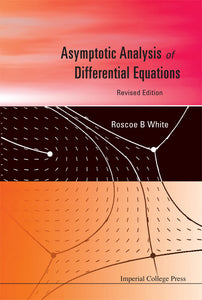# Asymptotic Analysis Of Differential Equations (Revised Edition)

Roscoe B White

Format: Print Book

ISBN: 9781848166073

• \$119.35
Unit price per
Tax included.

The book gives the practical means of finding asymptotic solutions to differential equations, and relates WKB methods, integral solutions, Kruskal-Newton diagrams, and boundary layer theory to one another. The construction of integral solutions and analytic continuation are used in conjunction with the asymptotic analysis, to show the interrelatedness of these methods. Some of the functions of classical analysis are used as examples, to provide an introduction to their analytic and asymptotic properties, and to give derivations of some of the important identities satisfied by them. The emphasis is on the various techniques of analysis: obtaining asymptotic limits, connecting different asymptotic solutions, and obtaining integral representation.

Format: Hardcover
No of Pages: 432
Imprint: Imperial College Press
Publication date: 20100816